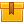# Introduction to Megohmmeters

None.

## Description

This is the first lesson in the iKNOW® Ammeters, Meggers, and Wheatstone Bridge Library. This lesson explains Ohm??s Law and how it is used when analyzing test results. The basic components, uses, and functions of a megohmmeter are described. Insulation and causes of insulation damage are also covered.

## Objectives

Explain the formula for Ohm??s Law. Explain how a working knowledge of Ohm??s Law can be helpful when analyzing test results. Describe, and give an example of, the use of a megger. List the causes of damaged insulation. Describe the causes and effects of low resistance readings. Identify the basic components of a typical megger. Identify the switches on a digital megger. Define the function of a megger.

## ContentThis is the first lesson in the iKNOW™ Ammeters, Meggers, and Wheatstone
Bridge Library. This lesson explains Ohm’s Law and how it is used when
analyzing test results. The basic components, uses, and functions of a
megohmmeter are described. Insulation and causes of insulation damage
are also covered.

•Introduction to Megohmeters2013

0
0 Reviews